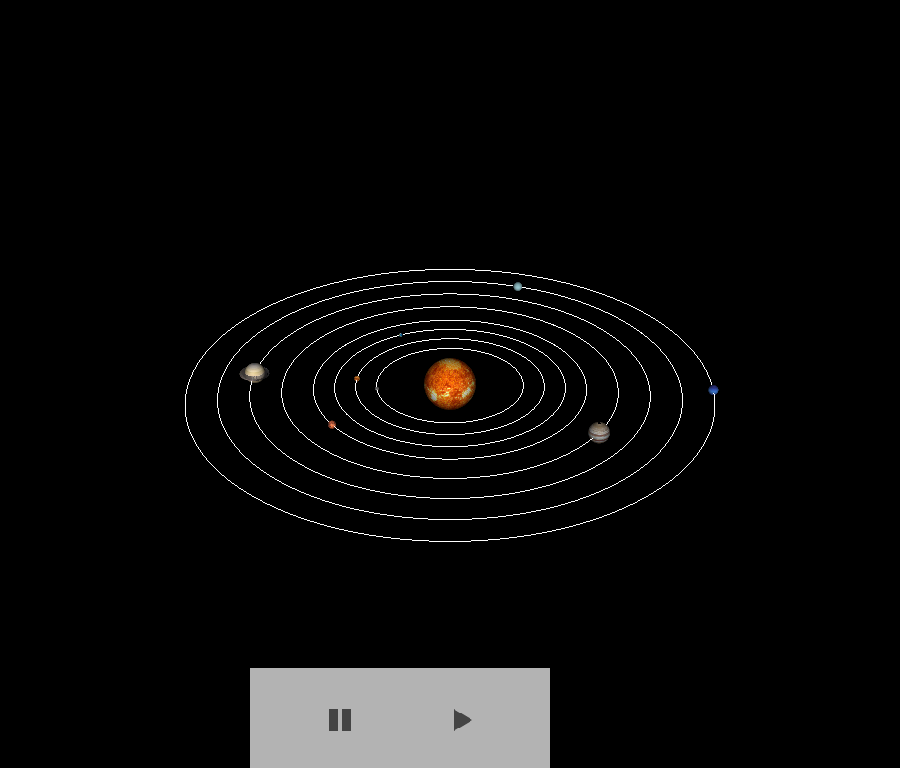# Solar System Animation¶

In this tutorial, we will create an animation of the solar system using textured spheres. We will also show how to manipulate the position of these sphere actors in a timer_callback function to simulate orbital motion.

```import numpy as np
from fury import window, actor, utils, io
import itertools
```

Create a scene to start.

```scene = window.Scene()
```

Load in a texture for each of the actors. To do this, we will create a function called `init_planet`, which will initialize each planet actor given its corresponding filename and actor name. It will also add each actor to the scene that has already been created.

```planet_filenames = ["8k_mercury.jpg", "8k_venus_surface.jpg",
"1_earth_8k.jpg", "8k_mars.jpg", "jupiter.jpg",
"8k_saturn.jpg", "8k_saturn_ring_alpha.png",
"2k_uranus.jpg", "2k_neptune.jpg",
"8k_sun.jpg"]
fetch_viz_textures()

def init_planet(filename):
"""Initialize a planet actor.

Parameters
----------
filename : str
The filename for the corresponding planet texture.

Returns
-------
planet_actor: actor
The corresponding sphere actor with texture applied.
"""
planet_actor = actor.texture_on_sphere(planet_image)
return planet_actor
```

Out:

```Dataset is already in place. If you want to fetch it again please first remove the folder /Users/koudoro/.fury/textures
```

Use the `map` function to create actors for each of the texture files in the `planet_files` list. Then, assign each actor to its corresponding actor in the list.

```planet_actor_list = list(map(init_planet, planet_filenames))

mercury_actor = planet_actor_list
venus_actor = planet_actor_list
earth_actor = planet_actor_list
mars_actor = planet_actor_list
jupiter_actor = planet_actor_list
saturn_actor = planet_actor_list
saturn_rings_actor = planet_actor_list
uranus_actor = planet_actor_list
neptune_actor = planet_actor_list
sun_actor = planet_actor_list

# Rotate this actor to correctly orient the texture
utils.rotate(jupiter_actor, (90, 1, 0, 0))
```

Next, change the positions and scales of the planets according to their position and size within the solar system (relatively). For the purpose of this tutorial, planet sizes and positions will not be completely accurate.

```sun_actor.SetScale(5, 5, 5)
mercury_actor.SetScale(0.4, 0.4, 0.4)
venus_actor.SetScale(0.6, 0.6, 0.6)
earth_actor.SetScale(0.4, 0.4, 0.4)
mars_actor.SetScale(0.8, 0.8, 0.8)
jupiter_actor.SetScale(2, 2, 2)
saturn_actor.SetScale(2, 2, 2)
saturn_rings_actor.SetScale(3, 0.5, 3)
uranus_actor.SetScale(1, 1, 1)
neptune_actor.SetScale(1, 1, 1)

mercury_actor.SetPosition(7, 0, 0)
venus_actor.SetPosition(9, 0, 0)
earth_actor.SetPosition(11, 0, 0)
mars_actor.SetPosition(13, 0, 0)
jupiter_actor.SetPosition(16, 0, 0)
saturn_actor.SetPosition(19, 0, 0)
saturn_rings_actor.SetPosition(19, 0, 0)
uranus_actor.SetPosition(22, 0, 0)
neptune_actor.SetPosition(25, 0, 0)
```

Define the gravitational constant G, the orbital radii of each of the planets, and the central mass of the sun. The gravity and mass will be used to calculate the orbital position, so multiply these two together to create a new constant, which we will call miu.

```r_mercury = 7
r_venus = 9
r_earth = 11
r_mars = 13
r_jupiter = 16
r_saturn = 19
r_uranus = 22
r_neptune = 25

g_exponent = np.float_power(10, -11)
g_constant = 6.673*g_exponent

m_exponent = np.power(10, 30)
m_constant = 1.989*m_exponent

miu = m_constant*g_constant
```

Let’s define two functions that will help us calculate the position of each planet as it orbits around the sun: `get_orbit_period` and `get_orbital_position`, using the constant miu and the orbital radii of each planet.

```def get_orbit_period(radius):

return (x, y)
```

Let’s change the camera position to visualize the planets better.

```scene.set_camera(position=(-20, 60, 100))
```

Next, create a ShowManager object. The ShowManager class is the interface between the scene, the window and the interactor.

```showm = window.ShowManager(scene,
size=(900, 768), reset_camera=False,
order_transparent=True)
```

Next, let’s focus on creating the animation. We can determine the duration of animation with using the `counter`. Use itertools to avoid global variables.

```counter = itertools.count()
```

To track and visualize the orbital paths of the planets, we will create several new variables to map each planet’s orbits using `actor.dots`. It is important to define the track variable for each planet as global, allowing it to be used within the `timer_callback` function. To do this, create a function called `get_orbit_actor`.

```def get_orbit_actor(orbit_points):
orbit_actor = actor.dots(orbit_points, color=(1, 1, 1),
opacity=1, dot_size=1)
return orbit_actor
```

All of the planets will have the same initial positions, so assign each of those to the positions variables for each planet. These variables will be updated within the `timer_callback` function. Initialize and add the orbit actors into the scene. Also initialize the track variables for each planet.

```orbit_points = np.zeros((1000, 3), dtype='f8')

mercury_orbit_actor = get_orbit_actor(orbit_points)
positions_mercury = utils.vertices_from_actor(mercury_orbit_actor)

venus_orbit_actor = get_orbit_actor(orbit_points)
positions_venus = utils.vertices_from_actor(venus_orbit_actor)

earth_orbit_actor = get_orbit_actor(orbit_points)
positions_earth = utils.vertices_from_actor(earth_orbit_actor)

mars_orbit_actor = get_orbit_actor(orbit_points)
positions_mars = utils.vertices_from_actor(mars_orbit_actor)

jupiter_orbit_actor = get_orbit_actor(orbit_points)
positions_jupiter = utils.vertices_from_actor(jupiter_orbit_actor)

saturn_orbit_actor = get_orbit_actor(orbit_points)
positions_saturn = utils.vertices_from_actor(saturn_orbit_actor)

uranus_orbit_actor = get_orbit_actor(orbit_points)
positions_uranus = utils.vertices_from_actor(uranus_orbit_actor)

neptune_orbit_actor = get_orbit_actor(orbit_points)
positions_neptune = utils.vertices_from_actor(neptune_orbit_actor)

mercury_track = []
venus_track = []
earth_track = []
mars_track = []
jupiter_track = []
saturn_track = []
uranus_track = []
neptune_track = []
```

Define two new functions to use in `timer_callback` to update the planet positions and their tracks to visualize their orbit: `update_track` and `update_planet_position`.

```def update_planet_position(r_planet, planet_actor, planet_track, cnt):
pos_planet = get_orbital_position(r_planet, cnt)
planet_actor.SetPosition(pos_planet, 0, pos_planet)
planet_track.append([pos_planet, 0, pos_planet])
return pos_planet

def update_track(positions_planet, planet_track, planet_orbit_actor):
positions_planet[:] = np.array(planet_track)
utils.update_actor(planet_orbit_actor)
```

Define the `timer_callback` function, which controls what events happen at certain times, using the counter. Redefine the position of each planet actor using `get_orbital_position,` assigning the x and y values of each planet’s position with the newly calculated ones. Append each new planet position to its corresponding track array.

```def timer_callback(_obj, _event):
cnt = next(counter)
showm.render()

global mercury_track, venus_track, earth_track, mars_track, jupiter_track
global saturn_track, uranus_track, neptune_track

update_planet_position(r_mercury, mercury_actor, mercury_track, cnt)

update_planet_position(r_venus, venus_actor, venus_track, cnt)

update_planet_position(r_earth, earth_actor, earth_track, cnt)

update_planet_position(r_mars, mars_actor, mars_track, cnt)

update_planet_position(r_jupiter, jupiter_actor, jupiter_track, cnt)

pos_saturn = update_planet_position(r_saturn, saturn_actor, saturn_track,
cnt)
saturn_rings_actor.SetPosition(pos_saturn, 0, pos_saturn)

update_planet_position(r_uranus, uranus_actor, uranus_track, cnt)

update_planet_position(r_neptune, neptune_actor, neptune_track, cnt)

if cnt == 999:
update_track(positions_mercury, mercury_track, mercury_orbit_actor)

update_track(positions_venus, venus_track, venus_orbit_actor)

update_track(positions_earth, earth_track, earth_orbit_actor)

update_track(positions_mars, mars_track, mars_orbit_actor)

update_track(positions_jupiter, jupiter_track, jupiter_orbit_actor)

update_track(positions_saturn, saturn_track, saturn_orbit_actor)

update_track(positions_uranus, uranus_track, uranus_orbit_actor)

update_track(positions_neptune, neptune_track, neptune_orbit_actor)

if cnt == 1500:
showm.exit()
```

Watch the planets orbit the sun in your new animation!

```showm.initialize()